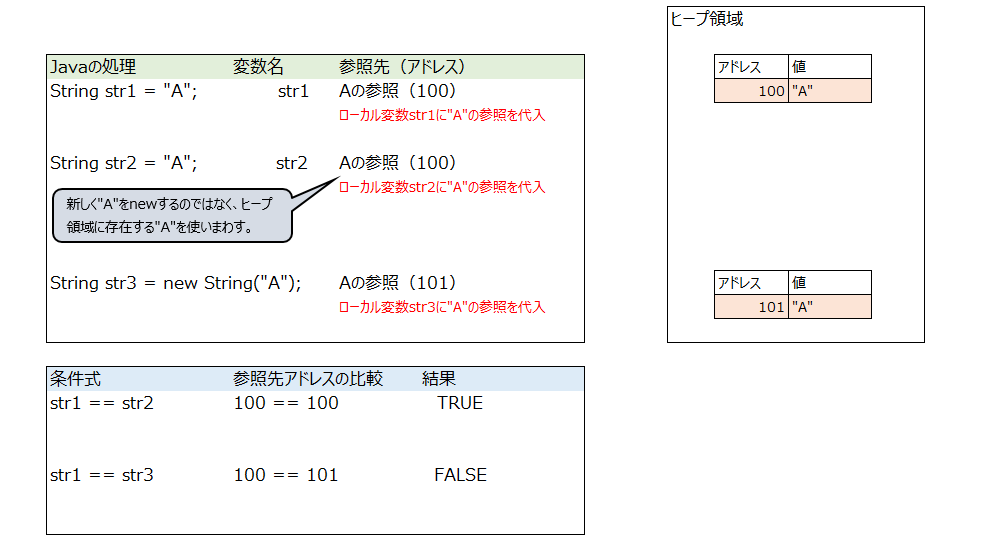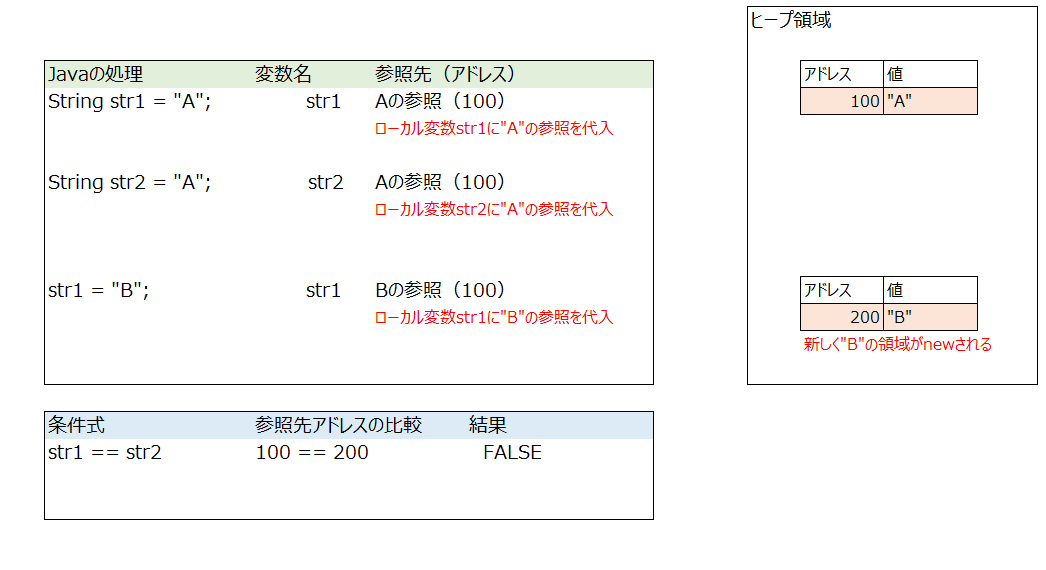# Let's dig deeper into String type comparison

Organized the comparison of String type values.

## Equal comparison method

1. == -For primitive types, compare values. -In the object type, the reference values of the objects are compared and it is judged whether the objects are the same.

2. equals() It can be used only in the object type, and the contents of the objects are compared to determine whether the objects have the same value.

• Equals is defined in the Object class. Override in String class.

In other words, Strng type is a reference type object, so you can use equals.

## Verification (Is it really impossible to compare with ==)

#### `StringSample.java`

``````
String str1 = "A";
String str2 = "A";
String str3 = new String("A");
System.out.println(str1.hashCode());
System.out.println(str2.hashCode());
if(str1 == str2) {
System.out.println("TRUE");
}else {
System.out.println("FALSE");
}
if(str1 == str3) {
System.out.println("TRUE");
}else {
System.out.println("FALSE");
}
``````

## result

``````100
100
TRUE
``````

It became TRUE. Is it okay to compare the String type with ==?

# Investigation

A value is created in the heap area when the String type is initialized, but it seems that it will be reused if the same value is used.

• If new, a new area will be created.

# I tried to illustrateTo save memory, use the area (address = 100) where the value "A" matches without new.

• Reference value: Memory address of the reference destination

## Behavior when changing the value of StringWhen you rewrite the value of a variable, the reference source value (address = 100) is not changed, but the new area of "B" is new and the reference destination is changed. (Changed the reference address from 100 to 200)

• Since String is immutable, the value of the reference destination cannot be changed.

# What is the strings object equals doing

#### `String.class`

``````
private final char value[];

public boolean equals(Object anObject) {
if (this == anObject) {
return true;
}
if (anObject instanceof String) {
String anotherString = (String)anObject;
int n = value.length;
if (n == anotherString.value.length) {
char v1[] = value;                //Value of own object
char v2[] = anotherString.value;  //Value of the compared object
int i = 0;
while (n-- != 0) {
if (v1[i] != v2[i])           //Decompose String into char and compare values
return false;
i++;
}
return true;
}
}
return false;
}
``````

The String object is decomposed into char [] and the values are compared one by one.

# Conclusion

If the String object has the same value to save memory, the reference value (reference address) may be the same for different local variables, but it is not guaranteed, so compare with equals.